# Percentage MCQ for Competitive Exams

Percentage MCQ Questions with Solution for Competitive Exams. Important question answer from the precious year exam question paper of SSC CGL, CPO, CSL and other govt jobs examinations. The Percentage MCQ are for the English Medium Students and Jobs aspirants.

## Basic Concept of Percentage

Percentage is always relative to 100. For example, a student score 64 numbers, Out of 100. That means he get 64% Marks.
Method =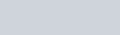$\dfrac{\text{ Obtained marks}}{\text{Total Marks}}$ x 100

% Marks =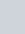$\dfrac{64}{100}$ x 100 = 64%

If someone score 24 Marks, out of total 50 marks – % Marks scored by him =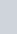$\dfrac{24}{50}$ x 100 = 48%

### Percentage MCQ Questions on Basic Concept

Q. 1: If x % of y % of 80 is the same as 25% of 900, then the value of xy is :
a) 30100
b) 32500
c) 28125
d) 34200

Ans : c) 28125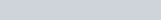$80\times \frac {y}{100} \times \frac{x}{100} = \frac{900 \times 25}{100}$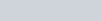$\frac {xy \times 80}{10000} = 9 \times 25$
=>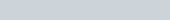$xy = \frac {9 \times 25 \times 10000}{80} =28125$

Q. 1: यदि 80 के y% का x%, 900 के 25% के समान है, तो xy का मान है:
a) 30100
b) 32500
c) 28125
d) 34200

Ans : c) 28125$80\times \frac {y}{100} \times \frac{x}{100} = \frac{900 \times 25}{100}$$\frac {xy \times 80}{10000} = 9 \times 25$
=>$xy = \frac {9 \times 25 \times 10000}{80} =28125$

Q.2: If 8% of x = 4% of y, then 20% of x is :
a) 10% of y
b) 16% of y
c) 40% of y
d) 80% of y

Ans : a) 10% of y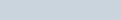$x \times \frac {8}{100} = y \times \frac {4}{100}$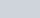$x= \frac y2$
20% of x is =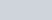$\frac {20}{100} \text { of } \frac y2$
=>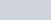$\frac{10}{100} \text{ of } y$= 10% of y

Q.3: In a village 30% of the population is literate. If the total population of the village is 6600, then the number of illiterate :
a) 1980
b) 4620
c) 2200
d) 3280

Ans : b) 4620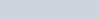$\frac {6600 \times 70}{100} = 4620$

Q.4: When 75 is added to 75% of a number, the answer is the number itself. Find 40% of that number :
a) 100
b) 80
c) 120
d) 160

Ans : c) 120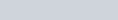$x \times \frac {75}{100} + 75 = x$
=>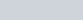$x - \frac {3x}{4} = 75$
=>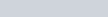$\frac x4 =75, x=300$
40% of 300 =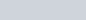$\frac {300 \times 40}{100} =120$

Q.5: In a big garden, 60% of the trees are coconut trees, 25% of the number of coconut trees are mango trees and 20% of the number of mango trees are apple trees. If the number of apple trees are 1500, then the number of trees in the garden is :
a) 48000
b) 50000
c) 51000
d) 45000

Ans : b) 50000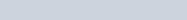$x \times \frac {60}{100} \times \frac{25}{100} \times \frac{20}{100} =1500$
=>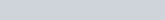$x = \frac{1500 \times 5 \times 4 \times 5}{3} = 50000$

Q.6: A box has 100 blue balls, 50 red balls, 50 black balls. 25% of blue balls and 50% of red balls are taken away. The percentage of black balls at present is :
a) 50%
b) 25%
c)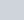$33\frac13$%
d) 40%

Ans : c)$33\frac13$ %
After taking away respective balls, remaining balls are = 75+25+50=150
Percentage of black balls =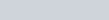$\frac {50}{150} \times 100 = 33\frac13$%

Q.7: The population of a village is 25,000. One fifth are females and the rest are males. 5% of males and 40% of females are uneducated. What percentage of the whole are educated?
a) 75%
b) 88%
c) 55%
d) 85%

Ans : b) 88%
Female one fifth = 5,000, Male = 25,000 -5,000 = 20000
Educated male = 95% of 20000 =19000
Educated Female = 60%of 5000 =3000
Total educated person = 22000
Required %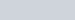$\frac {22000}{25000} \times100$=88%

Q.8: If 125% of x is 100, then x is :
a) 80
b) 150
c) 400
d) 125

Ans : a) 80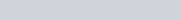$x \times \frac {125}{100} =100, => x =80$

Q.9: A team played 40 games in a season and won in 24 of them. In what per cent of games played did the team win?
a) 70%
b) 40%
c) 60%
d) 35%

Ans : c) 60%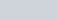$\frac {24}{40} \times 100$ = 60%

Q.10: The time duration of 1 hour 45 minutes is what per cent of a day?
a) 7.218%
b) 7.292%
c) 8.3%
d) 8.24%

Ans : b) 7.292%
Minutes = 60+45 = 105
Total Minutes in 24 hrs = 24×60
Required Percentage =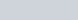$\frac{105}{24 \times 60} \times 100$ = 7.292%

Q.11: If 20% of A = 50% of B, then what per cent of A is B?
a) 30%
b) 40%
c) 25%
d) 15%

Ans : b) 40%
20% of A = 50%B
=> 2A = 5B
=> A =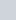$\frac {5B}{2}$
Let B is x% of A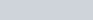$\frac {5B}{2} \times \frac{x}{100} = B$
=> x = 40%

### If x is Less/More than y by m% then y Exceeds/Lags than x

Q.12: If x earns 25% more than y. What percent less does y earn than x?
a) 16%
b) 10%
c) 20%
d) 25%

Ans : c) 20%
y =100, x =125
y earn 25 less than x
% less in respect of x =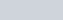$\frac {25}{125} \times 100$ = 20%
Short Trick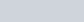$\frac {25}{100+25} \times 100$ = 20%

Q.13: B got 20% marks less than A. What percent marks did A got more than B?
a) 20%
b) 25%
c) 12%
d) 80%

Ans : b) 25%
A =100, B= 80
A got 20 marks more than B
Percentage more in respect of B =$\frac{20}{80} \times 100$= 25%
Direct Short Trick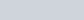$\frac {20}{100 - 20} \times 100$ = 25%

Q.14: Which number is 40% less than 90?
a) 36
b) 60
c) 50
d) 54

Ans : d) 54
60% of 90 =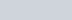$\frac {90 \times 60}{100} =54$

### Income, Expenditure, Salary and Wages : Percentage MCQ

Q.15 Rambabu donated 3% of his income to a charity and deposited 12% of the rest in bank. If now he has ₹ 12804, then his income was :
a) ₹ 17460
b)₹ 15000
c) ₹ 7500
d) ₹ 14550

Ans : b)₹ 15000
Let Rambabu salary ₹ A
Remaining after Donation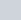$\frac {97A}{100}$
Remaining after deposit in bank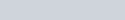$\frac{97A}{100} \times \frac {88}{100} = 12804$
=> A =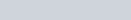$\frac{12804 \times 10000}{97 \times 88} = 15000$

Q.16: A man spends 75% of his income. His income is increased by 20% and he increased his expenditure by 10%. His savings is increased by :
a) 33 %
b) 50%
c) 25%
d) 10%

Ans : b) 50%
Income =100, Expenditure = 75, Saving 25
New Income = 120,
New Expenditure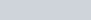$\frac {75 \times 110}{100} = 82.5$
New Saving = (120 – 82.5) = 37.5
Increase in saving = 37.5 – 25 = 12.5
% increase =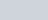$\frac {12.5 \times 100}{25}$ = 50%

Q.17: Two numbers are less than a third number by 30% and 37% respectively. How much per cent is the second number less than the first?
a) 10
b) 4
c) 3
d) 7

Ans : a) 10
3rd =100, 1st = 70, 2nd = 63
2nd number less than 1st = 70-63 = 7
% less from 1st number =$\frac {7}{70} \times 100$ = 10%

Q.18: Ram saves 14% of his salary while Shyam saves 22%. If both get the same salary and Shyam saves ₹ 1540, what is the savings of Ram?
a) ₹ 990
b) ₹ 980
c) ₹ 890
d) ₹ 880

Ans : b) ₹ 980
Let Ram and Shyam salary is ₹A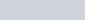$\frac {22 \times A}{100} =1540$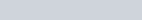$A= \frac{1540 \times 100}{22} = 7000$
Ram Saving =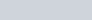$\frac {14 \times 7000}{100} =980$

Q.19: Tulsiram’s salary is 20% more than that of Kashyap. If Tulsiram saves ₹ 720 which is 4% of his salary, then Kashyap’s salary is :
a) ₹ 15,000
b) ₹ 12,000
c) ₹ 10,000
d) ₹ 22,000

Ans : a) ₹ 15,000
Let Tulsiram salary = A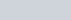$\frac {A \times 4}{100} = 720$
A = 18000
Kashyap Salary$\frac {100}{120} \times 18000 = 15000$

### Ration and Percentage MCQ

Q.20: The ratio of two numbers is 4: 5 when the first is increased by 20% and the second is decreased by 20%, the ratio of the resulting numbers is :
a) 4 : 5
b) 5 : 4
c) 5 : 6
d) 6 : 5

Ans : d) 6 : 5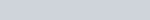$4x \times \frac{120}{100} : 5x \times {80}{100}$
= 12x = 12x => 6:5

Q.21: If 10% of m is the same as 20% of n, then m : n is equal to :
a) 2 : 1
b) 1 : 2
c) 1 : 10
d) 1 : 20

Ans : a) 2 : 1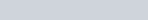$m \times \frac {10}{100} = n \times {20}{100}$$\frac mn = \frac {10}{5} = \frac 21$

### Alligation and Mixture (Percentage) Questions

Q.22: In one litre of a mixture of alcohol and water, water is 30%. The amount of alcohol that must be added to the mixture so that the part of water in the mixture becomes 15% is :
a) 1000 ml
b) 700 ml
c) 300 ml
d) 900 ml

Ans : a) 1000 ml
Alcohol = 700 ml and water = 300 ml
Let x ml of alcohol is mixed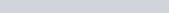${300}{1000+x} \times 100 =15$
=> 1000 + x = 2000
=> x = 1000 ml

Q.23: If 4 litres of water is evaporated on boiling from 12 litres of salt solution containing 7 percentage salt, the percentage of salt in the remaining solution is :
a) 10.5%
b) 11.5%
c) 12%
d) 13%

Ans : a) 10.5%
Salt =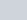$\frac {7 \times 12 }{100}$= 0.84 units
Water = 12 – 0.84 = 11.16 units
After evaporation, Percentage of salt =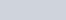$\frac {0.84}{8} \times 100$ = 10.5%

### Consumption and Expenditure : Percentage MCQ

Q.24: Price of milk has increased by 20%. To keep the expenditure unchanged, the present consumption is to be reduced by :
a) 20%
b) 18%
c) 10%
d)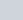$16 \frac 23$

Ans : d)$16 \frac 23$
Trick : Required percentage decrease =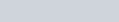$\frac{Increase}{100+Increase} \times 100$
Milk consumption percentage decrease =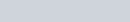$\frac {20}{100+20} \times 100 = 16\frac 23$%

Q.25: The price of a commodity rises from 6 per kg to7.50 per kg. If the expenditure cannot increase, the percentage of reduction in consumption is :
a) 15%
b) 20%
c) 25%
d) 30%

Ans : b) 20%
Percentage increase =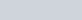$\frac{7.50 - 6}{6} \times 100$ = 25%
Percentage decrease in consumption =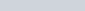${25}{125} \times 100$ = 20%

Q.26: If the duty on an article is reduced by 40% of its present rate, by how much percent must its consumption increase in order that the revenue remains unaltered?
a) 60%
b) 72%
c)$62\frac13$
d)$66\frac23$

Ans d)$66\frac23$
Required Increase =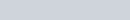$\frac{40}{100-40} \times 100 = 66\frac23$%

Q.27: If the price of a commodity is decreased by 20% and its consumption is increased by 20%, what will be the increase or decrease in the expenditure on the commodity?
a) 4% increase
b) 4% decrease
c) 8% decrease
d) 8% increase

Ans : b) 4% decrease
Let price = ₹100, and consumed = 100 unit
Expenditure : 100 x100 =₹ 10000
New price = 80, New consumption = 120 units
New Expenditure = 80 x 120 =₹ 9600
Decrease = ₹ 400
% percentage decrease =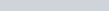${400 \times 100}{10000}$ = 4%

### Percentage MCQ based on Examinations and MarksObtained

Q. 28: A candidate who gets 20% marks in an examination, fails by 30 marks. But if he gets 32% marks, he gets 42 marks more than the minimum pass marks. Find the pass percentage of marks.
a) 52%
b) 20%
c) 25%
d) 12%

Ans : c) 25%
Let Maximum (full) marks is x.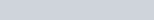$\frac {x \times 32}{100} - \frac{x \times 20}{100} = 30+42$
=> x =600
Minimum passing marks =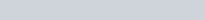$\frac{600 \times 20}{100} + 30 = 120+30 =150$
Pass percentage of marks =$\frac{150}{600} \times 100$ = 25%

Q. 28: A class has two sections, which contain 20 and 30 students. The pass percentage of these sections are 80% and 60% respectively. The pass percentage of whole class is :
a) 60%
b) 68%
c) 70%
d) 78%

Ans : b) 68%
Required % is =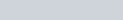$\frac{20 \times 80 + 30 \times 60}{50} = \frac {3400}{50}$= 68%

Q.29: In an examination, 19% students fail in Mathematics and 10% students fail in English. If 7% of all students fail in both subjects, then the number of students passed in both subjects is :
(a) 36 % of all students
(b) 64% of all students
(c) 71% of all students
(d) 78% of all students

Ans : (d) 78% of all students
Failed students in either one or two subjects = 19+10-7 = 22%
students passed in both subjects is : 100 -22 = 78%

Q.30: A student has to secure 40% marks to pass. He gets 90 marks and fails by 10 marks. Maximum marks are :
a) 200
b) 225
c) 250
d) 275

Ans : c) 250
Let maximum marks = x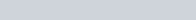$\frac {x \times 40}{100} = 90 +10, => x =250$

### Based on Tricks Net Increase or Decrease Percentage (MCQ)

Q.31: A number is first decreased by 20%. The decreased number is then increased by 20%. The resulting number is less than the original number by 20. Then the original number is :
a) 200
b) 400
c) 500
d) 600

Ans : d) 500
Trick : Net % change =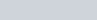$(A + B + \frac {AB} {100})$%
Effective percentage =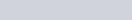$(-20 + 20 - \frac {20 \times 20}{100})$ = -4%
4% of number is 20
Number (100%) is 20 x 25 = 500

Q.32: The strength of a school increases and decreases in every alternate year by 10%. It started with increase in 2010. Then the strength of the school in 2013 as compared to that in 2010 was :
a) Increased by 8.9%
b) Decreased by 8.9%
c) Increased by 9.8%
d) Decrease by 9.8%

Ans : a) Increased by 8.9%
Let in 2010 students are 100
In 2011 increase 10% => 110
In 2012 decrease 10% =>110 – 11 = 99
In 2013 increase 10% =>99 +9.9 = 108.9
Increase in compare of 2010 = 108.9 – 100 = 8.9%

### Percentage Questions on Voters in an Election

Q.33: At an election there were two candidates. A candidate got 38% of votes and lost by 7200 number of votes. The total number of valid votes were :
a) 13000
b) 13800
c) 16200
d) 30000

Ans : d) 30000
Let valid vote = x
(62 – 38)% of x = 7200
=>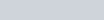$x \times \frac {24}{100} = 7200$
=> x = 30000

Q.34: 8% of the voters in an election did not cast their votes. In this election, there were only two candidates. The winner by obtaining 48% of the total votes defeated his contestant by 1100 votes. The total number of voters in the election was :
a) 21000
b) 23500
c) 22000
d) 27500

Ans : d) 27500
Let total vote 100
Number of vote polled = 100 – 8 = 92
Winner obtained 48
Loser obtained => 92 -48 =44
Difference of votes is 48 – 44 = 4 for 100 voters
For difference 1100 votes, total voters =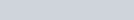$\frac {100}{4} \times 1100 =27500$

### Percentage MCQ on Depreciation and Population Increase

Q.35: The population of a village decreases at the rate of 20% per annum. If its population 2 years ago was 10,000 the present population is :
a) 4600
b) 6400
c) 7600
d) 6000

Ans : b) 6400
Present population is =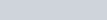$10000 (1-\frac{20}{100})^2$
=$10000 \times \frac45 \frac45 = 6400$

Q.36: The population of a village has increased annually at the rate of 25%. If at the end of 3 years it is 10,000, the population in the beginning of the first year was :
a) 5120
b) 5000
c) 4900
d) 4500

Ans : a) 5120
Population beginning of 1st year =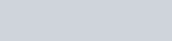$\dfrac{ \text{Population after 3 years}}{(1+\frac{Rate}{100})^{Time} }$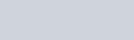$\dfrac {10000}{(1+\frac {25}{100})^3} = \dfrac {10000}{(\frac54)^3}$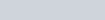$\frac {10000 \times 64}{125} = 5120$

### Reducing and Exceeding Prices : Percentage MCQ

Formula/ Trick: On increasing/decreasing the cost of a certain article by x%, a person can buy ‘a’ kg of article less/more in ‘y’ Rupee, then the increased/decreased cost of the commodity
New Price=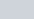$\frac {x \times y}{100 \times a}$
Old Price=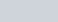$\frac{x \times y}{(100 \pm x) \times a}$

Q.37: If the price of apples is reduced by 25%, a customer gets 2 Kg more apples for ₹ 240, what is the reduced price per Kg?
a) 20 ₹ / Kg
b) 25 ₹ / Kg
c) 30 ₹ / Kg
d) 35 ₹ / Kg

c) 30 ₹ / Kg
Solve.      नया मूल्य =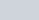$\frac{25 \times 240}{2 \times100}$ = 30 ₹ / Kg Ans
Formula/ Trick: On increasing/decreasing the cost of a certain article by x%, a person can buy ‘a’ kg of article less/more in ‘y’ Rupee, then the increased/decreased cost of the commodity
New Price=$\frac {x \times y}{100 \times a}$
Old Price=$\frac{x \times y}{(100 \pm x) \times a}$

Q.38: Due to 10% reduction in the price of tea, A person get 250 Gm of tea more than before in ₹ 270. The difference between the first price and the new price ( ₹ / Kg ) of tea ?
a) 12 ₹ / Kg
b) 14 ₹ / Kg
c) 17 ₹ / Kg
d) 21 ₹ / Kg

a) 12 ₹ / Kg
Solve.           New price =$\frac{10 \times270}{0.25 \times100}$ =108
Old Price =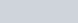$\frac{10 \times 270}{0.25 \times (100-10)}$ =120
difference = (120 – 108) = 12 ₹ / Kg Ans

Q.39: A reduction of 25% in the price of rice enables a person to buy 10 kg more rice for ₹ 600. The reduced per kg price of rice is :
a) ₹ 30
b) ₹ 25
c) ₹ 20
d) ₹ 15

Ans : d) ₹ 15
Reduced / New price =$\frac {25 \times 600}{100 \times 10} = 15$

Thanks for attempt Percentage MCQ questions for Competitive Exams.

### 4 thoughts on “Percentage MCQ for Competitive Exams”

1.Laharika

In 16th question, in the second point of the solution new income should be 120 not 125.

1.Corrected – It was typing error. Thanks a lot for pointing out the mistake.

2.DEEPAK

in question no. 32 it is clearly stated that “it starts with increase in 2010”
so
2010- 110
2011- 99
2012-108.9
2013-98.01

1.If you start from 2010 than calculate up to 2012. Because in that case strength of school in 2013 is the strength at the completion of 2012.